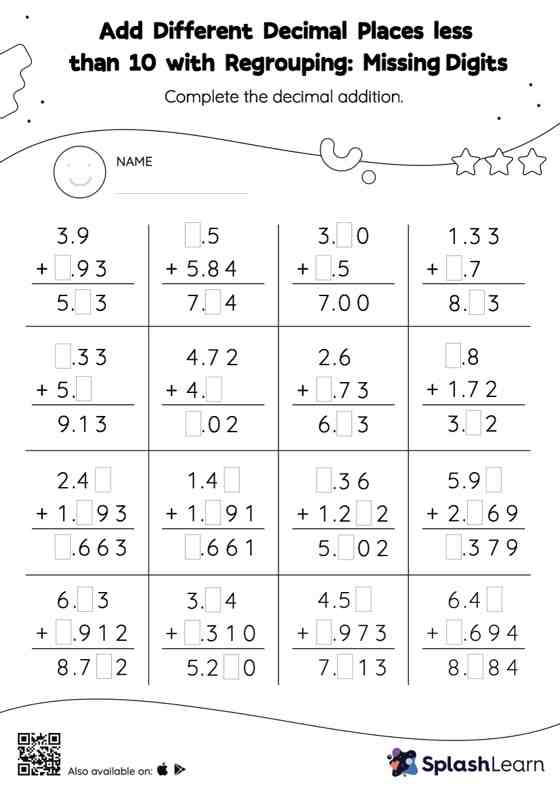# Add Different Decimal Places less than 10 with Regrouping: Missing Digits Worksheet

Home > Add Different Decimal Places less than 10 with Regrouping: Missing DigitsLearners hone their math skills with this add different decimal places less than 10 with regrouping worksheet. Students find the missing number while adding decimals by using the relationship between addition and subtraction. To reach the answer, they also use the relationship between ones, tenths, hundredths, thousandths, etc., To regroup the numbers in add different decimal places less than 10 with regrouping worksheet. This worksheet is about practicing the column method in which numbers are written one on top of another. In this method, students use their place value understanding to solve the problems.